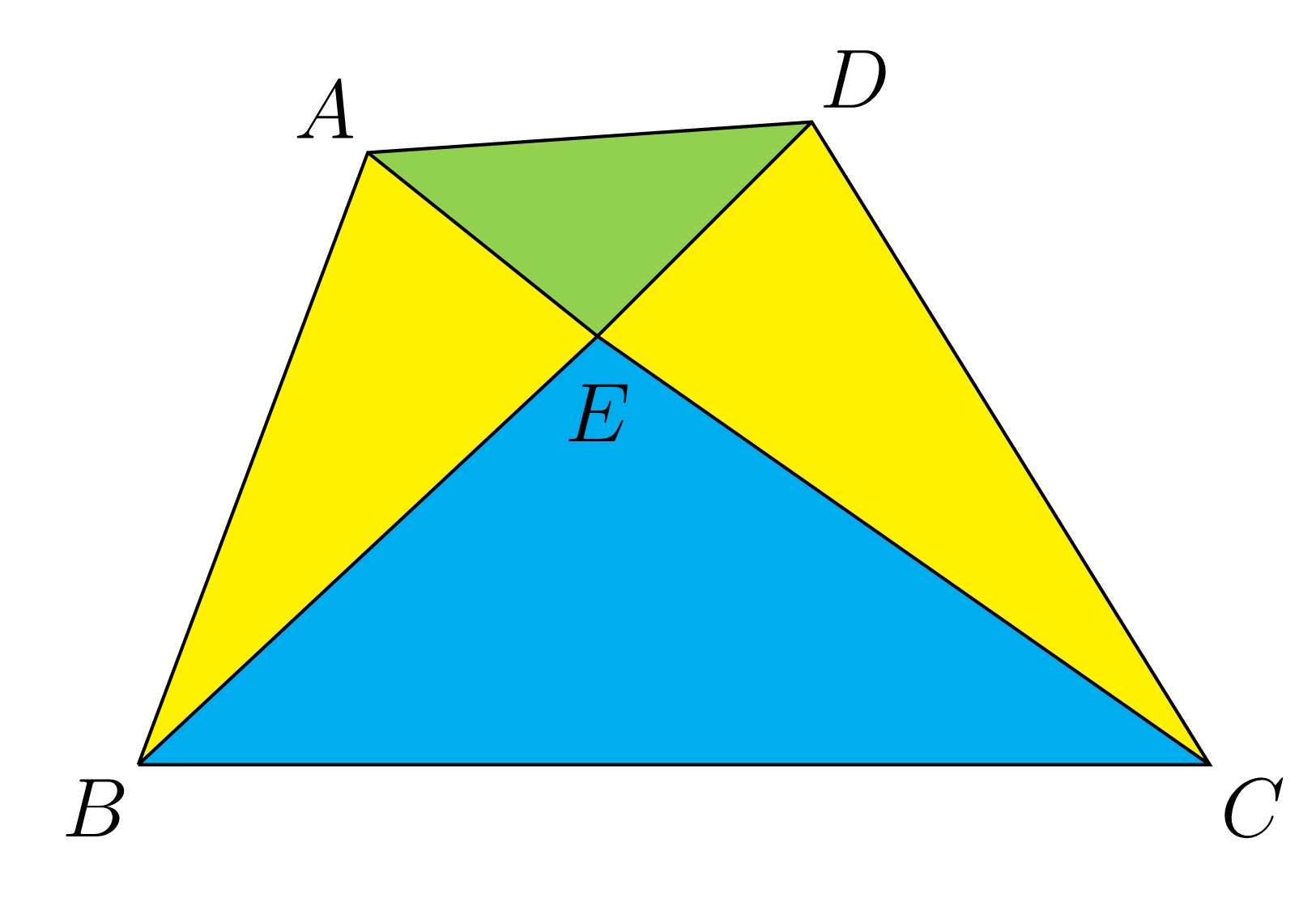# Question 1 of 101

Lines $AC$ and $BD$ divide the quadrilateral $ABCD$ into 4 triangles of different areas. Given that $BE:DE=2:1$ and $AE:CE=1:3$, find the ratio of the areas $\triangle ADE: \triangle BCE$.$3:7$
$2:5$
$1:4$